# ATOMIC STRUCTURE J J Thomsons plum pudding model

• Slides: 16
Download presentation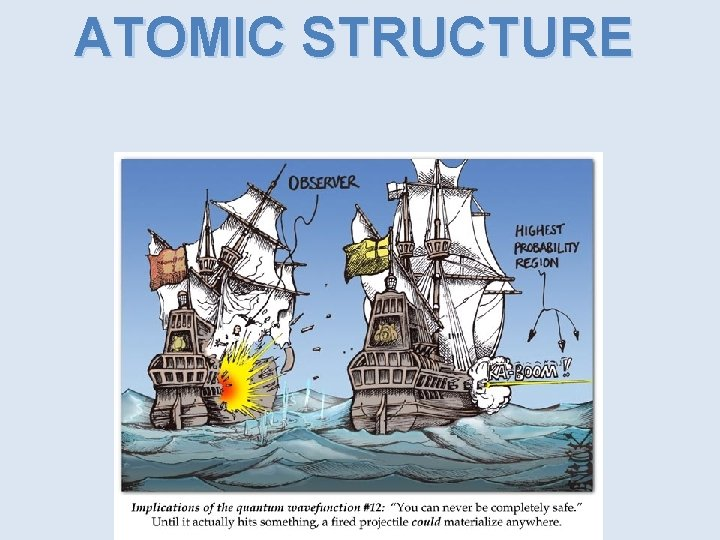ATOMIC STRUCTURE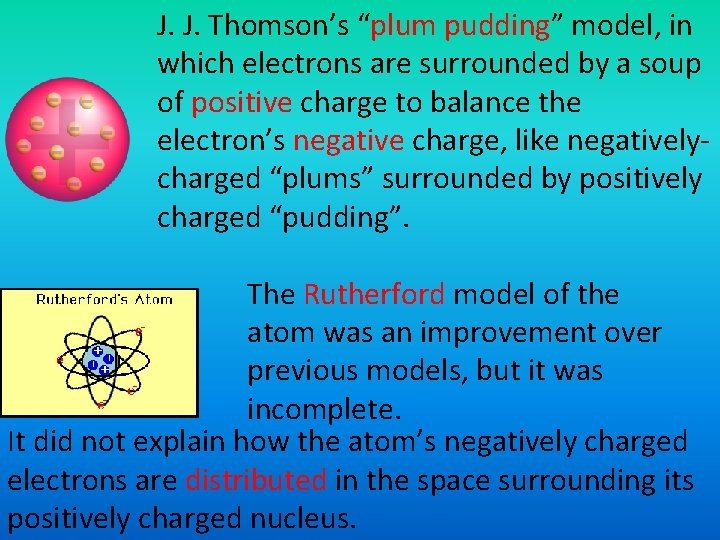J. J. Thomson’s “plum pudding” model, in which electrons are surrounded by a soup of positive charge to balance the electron’s negative charge, like negativelycharged “plums” surrounded by positively charged “pudding”. The Rutherford model of the atom was an improvement over previous models, but it was incomplete. It did not explain how the atom’s negatively charged electrons are distributed in the space surrounding its positively charged nucleus.The Dilemma of the Atom • Electrons outside the nucleus are attracted to the protons in the nucleus • Charged particles moving in curved paths lose energy • What keeps the atom from collapsing?In the early twentieth century, a new atomic model evolved as a result of investigations into the absorption and emission of light by matter. The studies revealed a relationship between light and an atom’s electrons. Before 1900, scientists thought light behaved solely as a wave. This belief changed when it was later discovered that light also has particle-like characteristics. A quick review of these wavelike properties follows.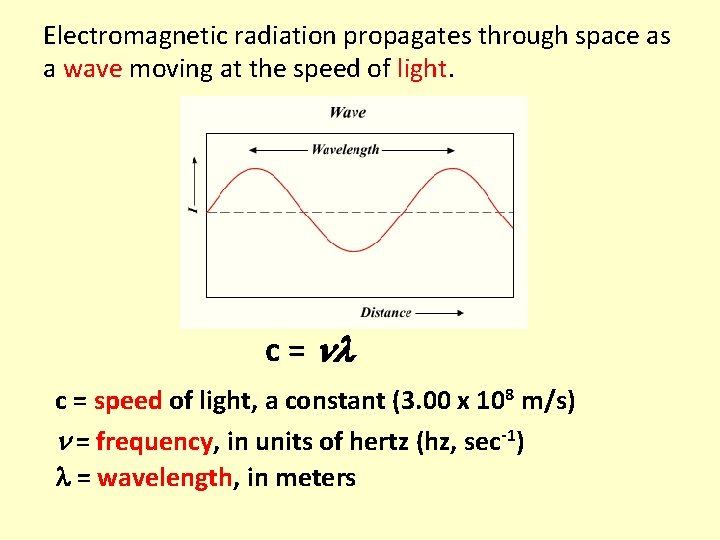Electromagnetic radiation propagates through space as a wave moving at the speed of light. c = speed of light, a constant (3. 00 x 108 m/s) = frequency, in units of hertz (hz, sec-1) = wavelength, in metersProperties of Waves Wavelength (λ) is the distance between identical points on successive waves. Amplitude is the vertical distance from the midline of a wave to the peak or trough.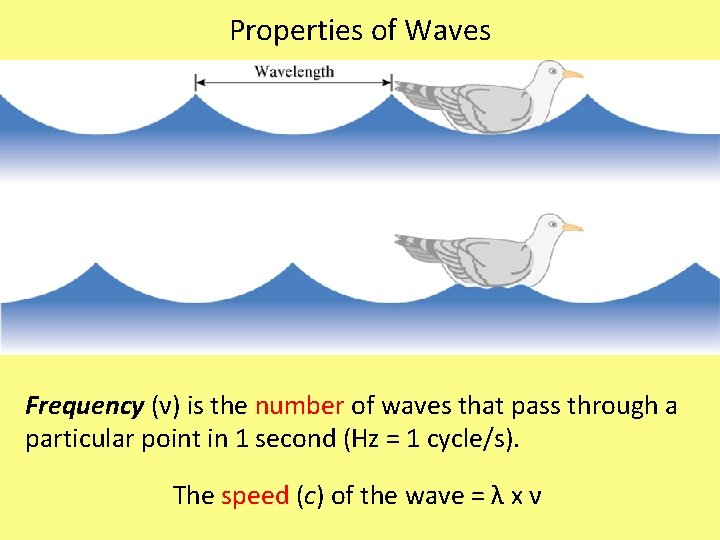Properties of Waves Frequency (ν) is the number of waves that pass through a particular point in 1 second (Hz = 1 cycle/s). The speed (c) of the wave = λ x ν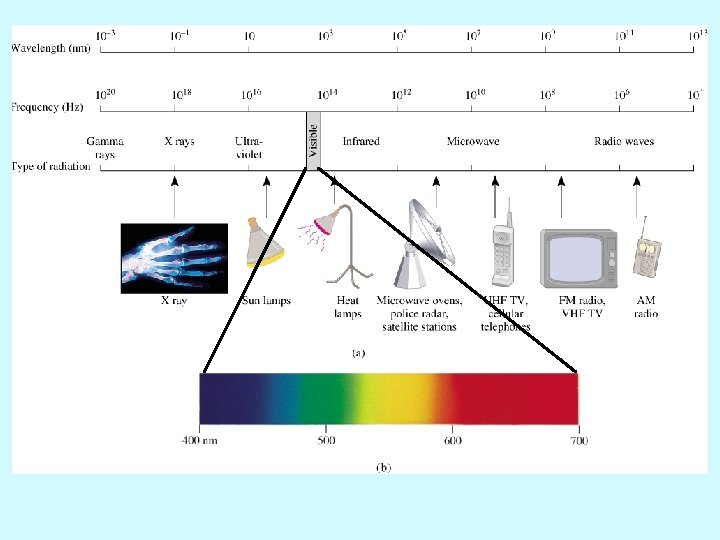In the early 1900 s, scientists conducted two experiments involving interactions of light and matter that could not be explained by the wave theory of light. 1900 – Max Planck – studied the light emitted by solid bodies heated to high temperatures. Found that the results could not be explained in terms of the wave theory of light. 1905 – Albert Einstein – Photoelectric effect – proposed that electromagnetic radiation is quantized.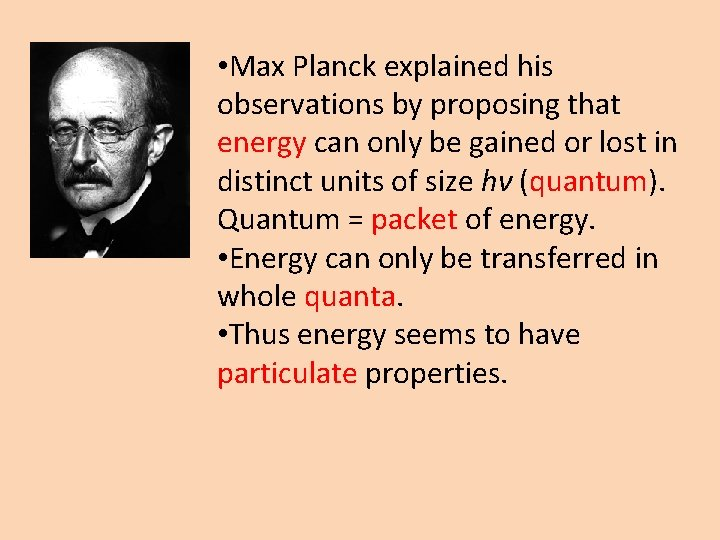• Max Planck explained his observations by proposing that energy can only be gained or lost in distinct units of size hν (quantum). Quantum = packet of energy. • Energy can only be transferred in whole quanta. • Thus energy seems to have particulate properties.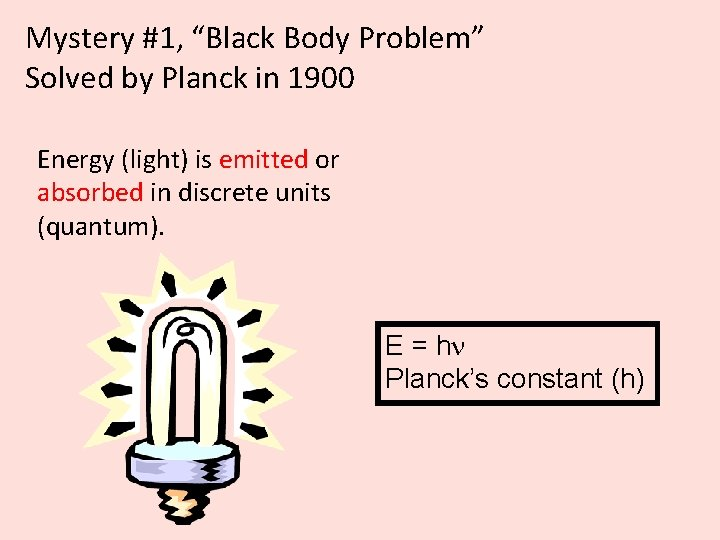Mystery #1, “Black Body Problem” Solved by Planck in 1900 Energy (light) is emitted or absorbed in discrete units (quantum). E = h Planck’s constant (h)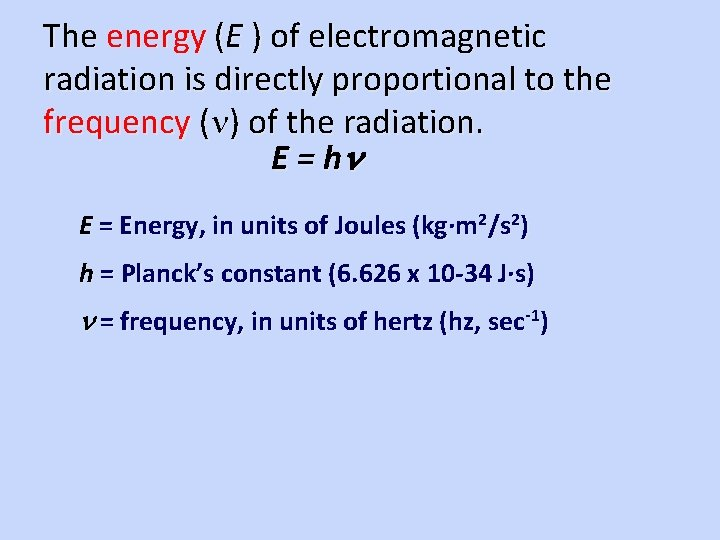The energy (E ) of electromagnetic radiation is directly proportional to the frequency ( ) of the radiation. E = h E = Energy, in units of Joules (kg·m 2/s 2) h = Planck’s constant (6. 626 x 10 -34 J·s) = frequency, in units of hertz (hz, sec-1)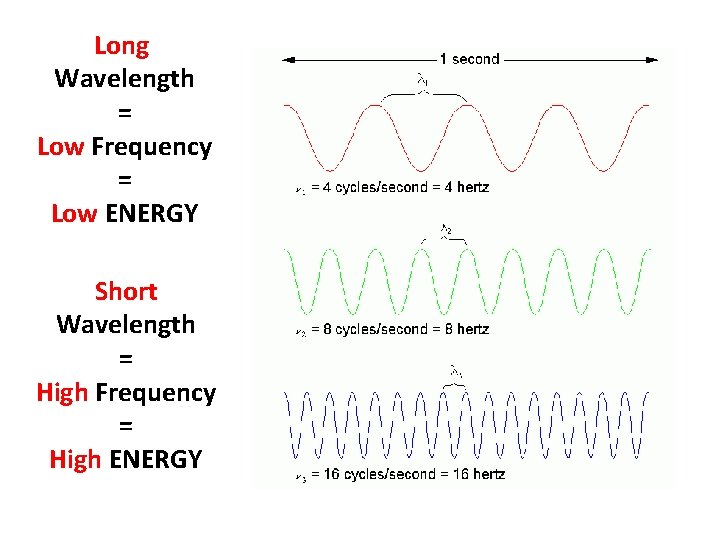Long Wavelength = Low Frequency = Low ENERGY Short Wavelength = High Frequency = High ENERGYPhotoelectric Effect • The photoelectric effect refers to the emission of electrons from a metal when light shines on the metal. Light may cause electrons to be emitted from an electrode in a photocell. Long wavelength light does not have enough energy to cause the electron to escape, regardless of its intensity. When light of a shorter wavelength (higher energy) light strikes the electrode, electrons are released. The amount of current produced depends on the intensity of the light and the energy of the escaping electrons depends on the wavelength of the light.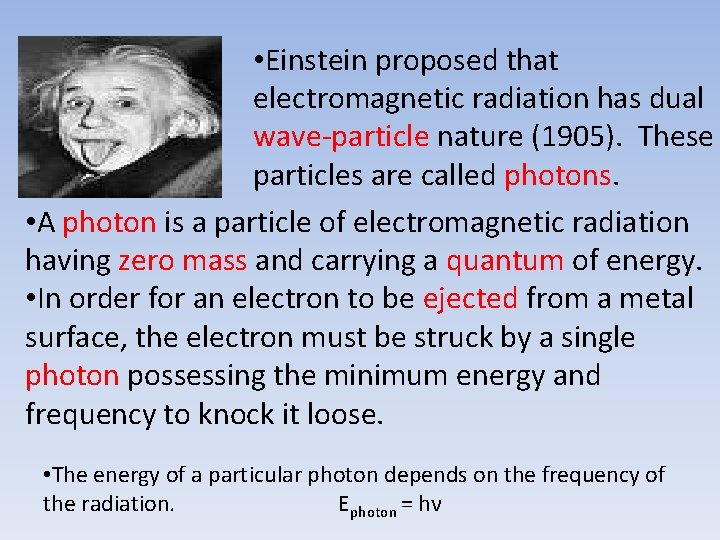• Einstein proposed that electromagnetic radiation has dual wave-particle nature (1905). These particles are called photons. • A photon is a particle of electromagnetic radiation having zero mass and carrying a quantum of energy. • In order for an electron to be ejected from a metal surface, the electron must be struck by a single photon possessing the minimum energy and frequency to knock it loose. • The energy of a particular photon depends on the frequency of the radiation. Ephoton = hνImportant conclusions from Planck and Einstein: Energy is quantized. It can occur only in discrete units called quanta. Electromagnetic radiation, which was previously thought to exhibit only wave properties, seems to show certain characteristics of particulate matter as well. This phenomenon is sometimes referred to as the dual nature of light.